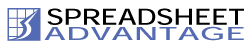Resource Links | Link to us | Sitemap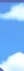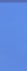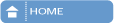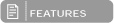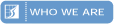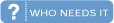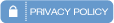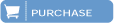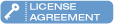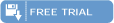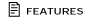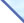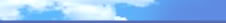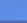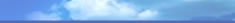Map

The Map tool produces a “map” of a worksheet. It shows which cells contain inputs and which contain formulae, and it shows the blocks of cells that all have the same formula. This is useful for getting an overall view of the structure of a worksheet, and it helps detect errors where, for example, a formula has not been correctly copied across a row, or where a hard-coded number has been placed in the middle of a row of formulae.

An example of a Map is shown below: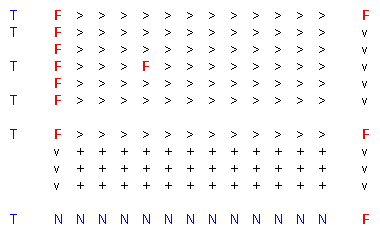For cells containing formulae: F Cell contains a formula > Cell contains the same formula as the cell on its left v Cell contains the same formula as the cell above it + Both > and v apply For cells containing inputs or constants: T Cell contains text (e.g. a label for a row or column) N Cell contains a number (e.g. a numerical user input)

The “F” in the middle of the 4th row indicates a change in the formulae across that row, which could be an error. Once this cell on the Map is selected, a special short-cut key will allow the user to jump between this cell and the corresponding cell in the spreadsheet being studied, allowing the user to conveniently investigate the apparent anomaly.

E-mail: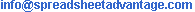| | | | | | |